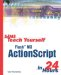I l @ ve RuBoard

 Look at the following bits of code and see whether you can determine the value of a before you read the solution: ` var a = 7; a++; a--; trace("a"); ` The result is 7. 1 is added to a and then 1 is taken away. ` var a = 7; a += 6 a *= 2; trace(a); ` The result is 26. a starts at 7, changes to 13 when 6 is added to it, and then is multiplied by 2 to get 26. ` var a = 7; var b = 3; a -= b; a++; trace(a); ` The result is 5. b is subtracted from a leaving 4. Then 1 is added to a . ` var a = 22; var b = 6; var c = 13; a = b + c; a -= 2; b++; trace(a); ` The result is 17. a starts off as 22, but the value is completely replaced by b + c , which is 19. Then 2 is subtracted from a , leaving 17. The b++ changes b to 5, but does not affect a at all.I l @ ve RuBoardSams Teach Yourself Flash MX ActionScript in 24 Hours
ISBN: 0672323850
EAN: 2147483647
Year: 2002
Pages: 272